# Scooter motor + encoder tunning.. Sudden motor rotation!

Hi.
I’m recently making mobile robot using scooter motors and incremental encoders.
There was a problem during tuning.

Test Environment
ubuntu 16.04
With a load of 60 kg on the robot
odrive V3.6-56V, and 0.4.10 is the latest firmware version.

Scooter motor

Encoder (HEDR-5421-EP111)
only a, b pulse
2100 cpr (include reduction ratio)
=> startup_encoder_offset_calibration = True (bool)

The variable I am touching while tuning
controller.config.pos_gain (fixed at 0.5)
controller.config.vel_gain = 0.014
controller.config.vel_integrator_gain = 0.007
controller.config.vel_limit = 5000
motor.config.current_control_bandwidth (fixed at 100)
encoder.config.bandwidth (fixed at 100)

Tunning step

python3 tunning.py

1. set parameter & set closed loop state
2. vel_setpoint -1000 ( my motor direction is “-1”)
3. 2 sec
4. vel_Setpoint 0
5. idle state

Symptom

``````In : dump_errors(odrv0)
axis0
axis: no error
motor: no error
encoder: no error
controller: no error
axis1
axis: Error(s):
ERROR_CONTROLLER_FAILED
motor: Error(s):
encoder: no error
controller: Error(s):
ERROR_OVERSPEED
``````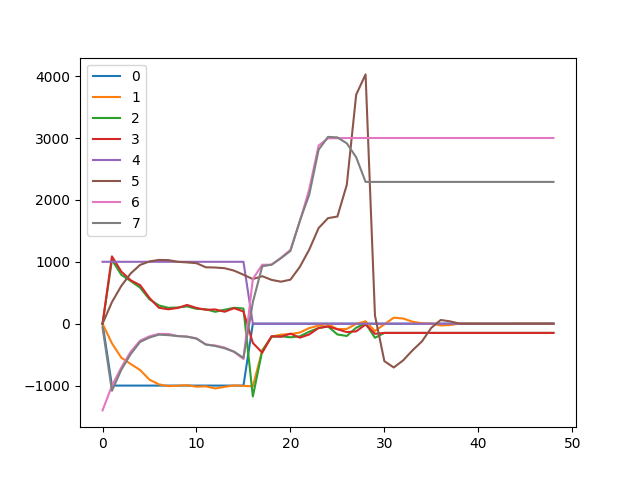``````start_liveplotter(lambda: [
odrv0.axis0.controller.vel_setpoint,
odrv0.axis0.encoder.vel_estimate,
odrv0.axis0.motor.current_control.Iq_setpoint*100.,
odrv0.axis0.motor.current_control.Iq_measured*100.,
odrv0.axis1.controller.vel_setpoint,
odrv0.axis1.encoder.vel_estimate,
odrv0.axis1.motor.current_control.Iq_setpoint*100.,
odrv0.axis1.motor.current_control.Iq_measured*100. ])
``````

It is done well once at first.
Repeatedly doing the same experiment.
The axis1 motor suddenly rotates as dangerously as above.
As you can see, axis1…iq_setpoint is very high.

After above situation, Parameter list
``````In : odrv0.axis1.motor
Out:
armed_state = 0 (int)
DC_calib_phB = -0.753932535648346 (float)
is_calibrated = True (bool)
error = 0x0010 (int)
DC_calib_phC = 0.43294408917427063 (float)
timing_log:
TIMING_LOG_FOC_CURRENT = 10486 (int)
TIMING_LOG_MEAS_L = 0 (int)
TIMING_LOG_FOC_VOLTAGE = 8882 (int)
TIMING_LOG_GENERAL = 0 (int)
TIMING_LOG_IDX_SEARCH = 0 (int)
TIMING_LOG_MEAS_R = 0 (int)
TIMING_LOG_ENC_CALIB = 8934 (int)
gate_driver:
drv_fault = 0 (int)
current_control:
overcurrent_trip_level = 33.75 (float)
v_current_control_integral_q = 0.0 (float)
v_current_control_integral_d = 0.0 (float)
final_v_alpha = -10.750458717346191 (float)
Iq_setpoint = 30.0 (float)
Ibus = 14.393915176391602 (float)
I_measured_report_filter_k = 1.0 (float)
p_gain = 0.055089570581912994 (float)
Id_measured = -0.6554780006408691 (float)
final_v_beta = 3.133859634399414 (float)
i_gain = 27.047452926635742 (float)
Iq_measured = 21.923112869262695 (float)
max_allowed_current = 30.375 (float)
config:
motor_type = 0 (int)
phase_inductance = 0.0005508956965059042 (float)
phase_resistance = 0.27047452330589294 (float)
inverter_temp_limit_lower = 100.0 (float)
requested_current_range = 25.0 (float)
current_control_bandwidth = 100.0 (float)
calibration_current = 10.0 (float)
current_lim = 30.0 (float)
pre_calibrated = True (bool)
pole_pairs = 15 (int)
inverter_temp_limit_upper = 120.0 (float)
resistance_calib_max_voltage = 4.0 (float)
direction = -1 (int)
get_inverter_temp()
thermal_current_lim = 139.79164123535156 (float)
current_meas_phC = -0.05028080940246582 (float)
phase_current_rev_gain = 0.012500000186264515 (float)
current_meas_phB = -0.0312504768371582 (float)

In : odrv0.axis1.encoder
Out:
set_linear_count(count: int)
error = 0x0000 (int)
interpolation = 0.5 (float)
phase = -1.2407751083374023 (float)
calib_scan_response = 1125.0 (float)
pos_estimate = 855.9981079101562 (float)
pos_cpr = 855.97509765625 (float)
hall_state = 5 (int)
config:
enable_phase_interpolation = True (bool)
calib_range = 0.019999999552965164 (float)
use_index = False (bool)
calib_scan_distance = 50.26548385620117 (float)
calib_scan_omega = 12.566370964050293 (float)
ignore_illegal_hall_state = False (bool)
mode = 0 (int)
offset_float = 0.14659374952316284 (float)
offset = -517 (int)
cpr = 2100 (int)
idx_search_unidirectional = False (bool)
pre_calibrated = True (bool)
bandwidth = 100.0 (float)
zero_count_on_find_idx = True (bool)
find_idx_on_lockin_only = False (bool)
count_in_cpr = 855 (int)
vel_estimate = 0.0 (float)
index_found = False (bool)

In : odrv0.axis1.config
Out:
lockin:
vel = 40.0 (float)
current = 10.0 (float)
accel = 20.0 (float)
finish_distance = 100.0 (float)
ramp_time = 0.4000000059604645 (float)
finish_on_vel = False (bool)
finish_on_distance = False (bool)
ramp_distance = 3.1415927410125732 (float)
finish_on_enc_idx = False (bool)
startup_sensorless_control = False (bool)
startup_encoder_offset_calibration = True (bool)
enable_step_dir = False (bool)
startup_encoder_index_search = False (bool)
counts_per_step = 2.0 (float)
step_gpio_pin = 7 (int)
dir_gpio_pin = 8 (int)
startup_motor_calibration = False (bool)
watchdog_timeout = 0.0 (float)
startup_closed_loop_control = False (bool)

In : odrv0.axis1.controller
Out:
set_pos_setpoint(pos_setpoint: float, vel_feed_forward: float, current_feed_forward: float)
vel_ramp_enable = False (bool)
error = 0x0001 (int)
current_setpoint = 0.0 (float)
vel_integrator_current = 0.0 (float)
move_to_pos(pos_setpoint: float)
config:
vel_gain = 0.014000000432133675 (float)
vel_limit = 5000.0 (float)
control_mode = 2 (int)
setpoints_in_cpr = False (bool)
vel_limit_tolerance = 1.2000000476837158 (float)
vel_integrator_gain = 0.009999999776482582 (float)
vel_ramp_rate = 10000.0 (float)
pos_gain = 0.5 (float)
vel_ramp_target = 0.0 (float)
vel_setpoint = 0.0 (float)
set_current_setpoint(current_setpoint: float)
move_incremental(displacement: float, from_goal_point: bool)
start_anticogging_calibration()
set_vel_setpoint(vel_setpoint: float, current_feed_forward: float)
pos_setpoint = 0.0 (float)
``````

Suspicious point
(After above situation)
Iq_setpoint = 30.0 (float) => I don’t touch any current_setpoint.
Is the current integral variable inside the controller too high?

Question

1. Is it simply a gain problem? Is my gain too high?
2. Why is the current target value raised?
3. Is there any other checkable variable?

Thank you.

Since your motor direction is -1, it is usually that Iq (setpoint and measured) are negative when the speed is positive. You can see this from 0s to 15s. Then when you asked it to stop it seems you got a problem with the encoder such that the commutation is wrong. The reason: The current is positive, so the speed should go towards negative, but it goes more positive instead (because direction is -1 like stated).

There are usually two main causes of encoder commutation error: mechanical slip of the encoder, or electrical noise. Please check for both of those.

1 Like

Ah. I understand what you said.

AXIS 1

In normal case, it looks good.
Since my direction is “-1”, so current is negative when vel_setpoint is positive.
(axis1’s vel_setpoint is 1000)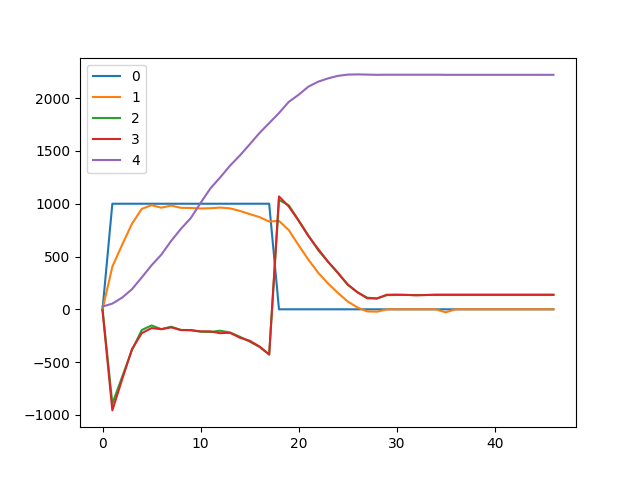This situation happens after several more attempts. As you said, it is weird.
After about 15 sec(?), when current is positive, also velocity goes positive high.
(it must be go to negative…)
But… i can’t see any slip on shadow_count. (am i right?)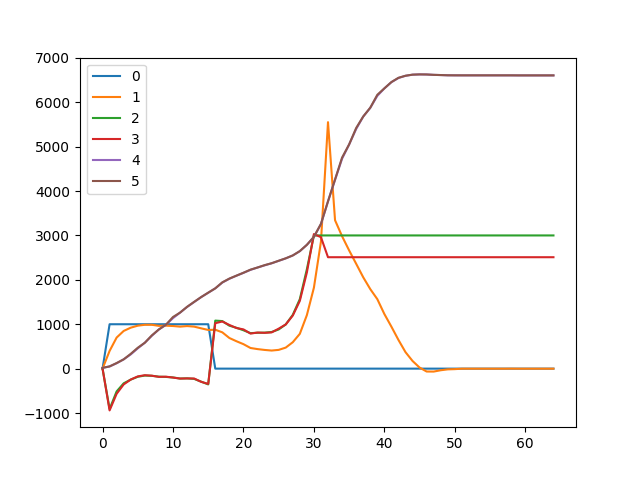``````start_liveplotter(lambda: [
odrv0.axis1.controller.vel_setpoint,
odrv0.axis1.encoder.vel_estimate,
odrv0.axis1.motor.current_control.Iq_setpoint*100.,
odrv0.axis1.motor.current_control.Iq_measured*100.,
odrv0.axis1.encoder.pos_estimate])
``````

but…I don’t know why iq_setpoint goes up anyway.

And… I think it does not seem to be a mechanical slip problem.
Because the encoder is mechanically very tightly fixed.
I’ll try to find reason that slip and electrical problem.

Hmm…

First i check my incremental encoder waveform.

5V, A, B

Axis 0,1 is similar waveform. Clear waveform.
and… hand turn motor.
(hmm. i don’t know this is right method. but it looks good.)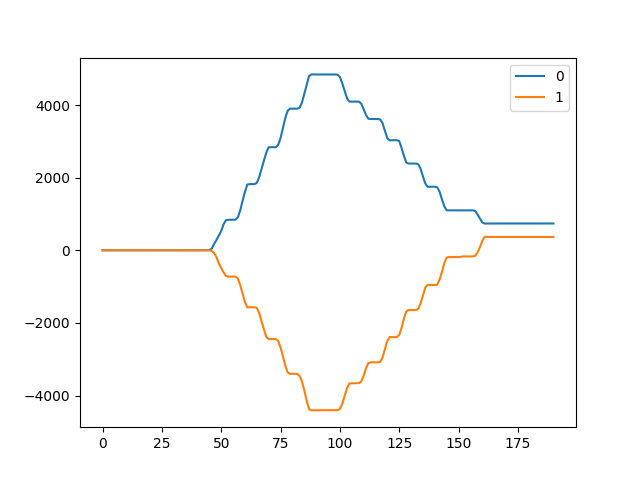There are two things that make me feel hard.

1. I know that the controller algorithm is not wrong.
2. It is difficult to reproduce symptoms because these symptoms occur occasionally.

hahaThere is no load on hoverboard motor in the air.
I see some strange symptom.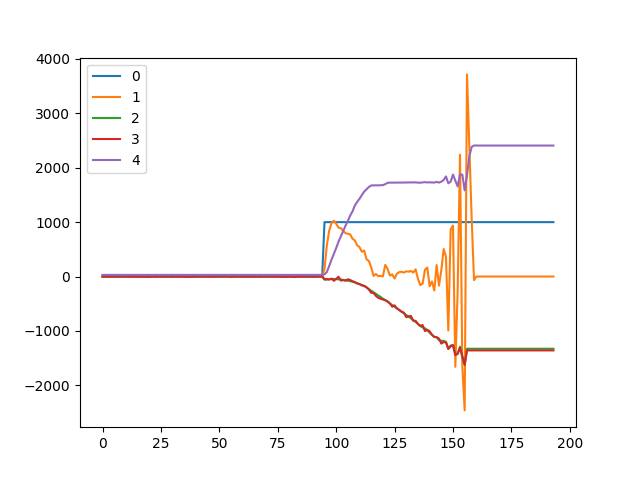start_liveplotter(lambda: [
odrv0.axis0.controller.vel_setpoint,
odrv0.axis0.encoder.vel_estimate,
odrv0.axis0.motor.current_control.Iq_setpoint100.,
odrv0.axis0.motor.current_control.Iq_measured
100.,

It looks like…

The target current value increases but hoverboard motor does not seem to operate.

After all about 150 (x axis), motor runaway.

What do you think the cause is? Any parameter modify suggestion? @madcowswe
The error is the same as above.

axis: Error(s):
ERROR_CONTROLLER_FAILED
motor: Error(s):
encoder: no error
controller: Error(s):
ERROR_OVERSPEED

Parameter in this situation

In : odrv0.axis0.encoder
Out:
index_found = False (bool)
set_linear_count(count: int)
phase = 0.12262439727783203 (float)
interpolation = 0.5 (float)
count_in_cpr = 308 (int)
hall_state = 6 (int)
pos_estimate = 2408.995361328125 (float)
error = 0x0000 (int)
config:
mode = 0 (int)
calib_range = 0.019999999552965164 (float)
enable_phase_interpolation = True (bool)
ignore_illegal_hall_state = False (bool)
calib_scan_distance = 50.26548385620117 (float)
offset = -534 (int)
pre_calibrated = True (bool)
use_index = False (bool)
calib_scan_omega = 12.566370964050293 (float)
cpr = 2100 (int)
offset_float = -0.2323906421661377 (float)
bandwidth = 100.0 (float)
zero_count_on_find_idx = True (bool)
find_idx_on_lockin_only = False (bool)
idx_search_unidirectional = False (bool)
vel_estimate = 0.0 (float)
pos_cpr = 308.97509765625 (float)
calib_scan_response = 1133.0 (float)

In : odrv0.axis0.motor
Out:
get_inverter_temp()
is_calibrated = True (bool)
current_control:
overcurrent_trip_level = 33.75 (float)
max_allowed_current = 30.375 (float)
v_current_control_integral_q = -3.9443492889404297 (float)
Id_measured = -0.3558006286621094 (float)
i_gain = 28.756166458129883 (float)
final_v_alpha = 3.618594169616699 (float)
final_v_beta = 2.030857563018799 (float)
Ibus = 2.9069974422454834 (float)
Iq_measured = -13.584692001342773 (float)
Iq_setpoint = -13.277606964111328 (float)
v_current_control_integral_d = 1.317560076713562 (float)
I_measured_report_filter_k = 1.0 (float)
p_gain = 0.054605428129434586 (float)
DC_calib_phB = -0.28327295184135437 (float)
current_meas_phC = 0.06704998016357422 (float)
armed_state = 0 (int)
DC_calib_phC = -1.1748133897781372 (float)
error = 0x0010 (int)
config:
pole_pairs = 15 (int)
motor_type = 0 (int)
requested_current_range = 25.0 (float)
phase_resistance = 0.28756165504455566 (float)
inverter_temp_limit_upper = 120.0 (float)
inverter_temp_limit_lower = 100.0 (float)
pre_calibrated = True (bool)
phase_inductance = 0.0005460542743094265 (float)
resistance_calib_max_voltage = 4.0 (float)
current_lim = 30.0 (float)
direction = -1 (int)
current_control_bandwidth = 100.0 (float)
calibration_current = 10.0 (float)
phase_current_rev_gain = 0.012500000186264515 (float)
thermal_current_lim = 134.62911987304688 (float)
current_meas_phB = -0.2603732943534851 (float)
timing_log:
TIMING_LOG_FOC_VOLTAGE = 6958 (int)
TIMING_LOG_IDX_SEARCH = 0 (int)
TIMING_LOG_ENC_CALIB = 7010 (int)
TIMING_LOG_GENERAL = 0 (int)
TIMING_LOG_MEAS_L = 0 (int)
TIMING_LOG_FOC_CURRENT = 7518 (int)
TIMING_LOG_MEAS_R = 0 (int)
gate_driver:
drv_fault = 0 (int)

In : odrv0.axis0.controller
Out:
set_current_setpoint(current_setpoint: float)
move_to_pos(pos_setpoint: float)
set_pos_setpoint(pos_setpoint: float, vel_feed_forward: float, current_feed_forward: float)
vel_integrator_current = 14.576169967651367 (float)
move_incremental(displacement: float, from_goal_point: bool)
vel_ramp_target = 0.0 (float)
start_anticogging_calibration()
vel_ramp_enable = False (bool)
vel_setpoint = 0.0 (float)
config:
vel_limit_tolerance = 1.2000000476837158 (float)
vel_limit = 3000.0 (float)
vel_integrator_gain = 0.0024999999441206455 (float)
vel_gain = 0.0005000000237487257 (float)
pos_gain = 0.5 (float)
control_mode = 2 (int)
setpoints_in_cpr = False (bool)
vel_ramp_rate = 10000.0 (float)
pos_setpoint = 30.9965877532959 (float)
error = 0x0001 (int)
set_vel_setpoint(vel_setpoint: float, current_feed_forward: float)
current_setpoint = 0.0 (float)

I also set “vel_limit_tolerance = 0”
it goes too high velocity oscillation.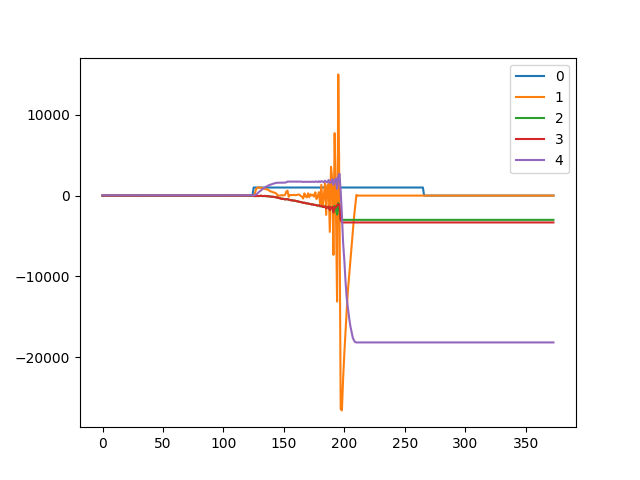Hi!

We are also trying to use ODrive for a mobile robot and are seeing similar strange behavior when using an optical encoder.

We got it to work pretty well by using hall encoders after upgrading to the newest firmware (older firmware had issues, possibly because of Z-interrupt on hall noise on the other motor-channel).
We put up a video of remote-control-by-smartphone here:

The resolution for controlling the motor on low speed is not good enough for autonomous driving with the hall encoders, and we were hoping to fix that by using optical encoders instead.

When using optical encoders (without index pulse), the position control without load seems to work ok sometimes.

Velocity control has this odd behaviour where the motor stops and the current rises very quickly at the same time:

Current control does not seem to be able to get the motor to move at all.

We have also seen the behaviour in velocity control mode where the motor suddenly rotates a few turns and slows down after a while. That seems similar to what has been discussed here earlier.

Does the motor controller simply not support this combination of motor, encoder and target velocity or is there some way to get this to work by changing the config?

It would be nice to have support for both hall and optical encoder at the same time to allow restarting without calibration for setups with no index signal.

`encoder.config.bandwidth = 1000` (this is the actual default)
`motor.config.current_control_bandwidth = 1000` (also default)

I started out with “encoder.config.bandwidth = 1000” and changed it to 500 to se if that made any difference. It did not seem to matter much in this case, and I apparently forgot to change it back.

The “Hoverboard Guide” mentioned that you should reduce controller bandwidth for high inductance motors. I have tried the default, but that doesn’t work either.

I did some tests with an industrial driver yesterday (iPOS8020 BX-CAN) and had the same problems. So this is apparently not a ODrive specific issue.

It turns out that there is an insane amount of EMI on the A & B encoder signals when current is running through the motor.
This is the noise from just running encoder calibration:

When a couple of amps are running through the motor, the oscilloscope only shows noise between A and B.

I got rid of some of the noise by separating the motor and encoder wires, but there is still quite a bit of noise left.

I also emailed the manufacturer and they suggested changing the order of the motor phase connector cables. Not sure why this would help with the noise, but I will give it a shot after my Christmas break.

Pretty surprised that the ODrive managed to filter out the encoder noise so well before the breaking point.

1 Like

Editing a response with regards to sam_dyno’s post…

Wow, yeah, that’ll do it. Did you end up fixing this? ODrive does well with white noise, as it has a velocity observer for zero-lag filtering. It’s relatively simple, but robust to gaussian noise.

Hi sam_dyno, did you get to the bottom of this issue? As it happens, I have purchased two motors that look identical to yours and I am having similar problems. I got them to run nicely using the hall sensors on a VESC but having real issues using the optical encoder on ODRIVE. Any further advice on overcoming this issue would be welcome! Thanks.

Just wanted to point out that you might see this kind of behaviour if your encoder is slipping. This could be caused by a number of issues such as:

• encoder not securely mounted,
• encoder is not mounted directly on the motor output shaft and there is slip somewhere else,
• the encoder signal is being distorted, causing it to miss increments

All of these things are more likely to cause problems as more control effort is applied.